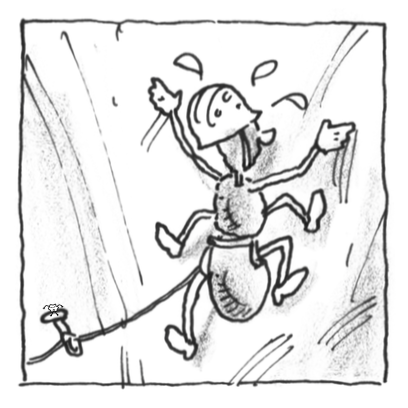### Home > APCALC > Chapter 12 > Lesson 12.1.1 > Problem12-9

12-9.

A bug is climbing up a wall. When the bug starts out, it has the energy to climb up quickly, but as time passes it tires, and climbs more and more horizontally on the wall, which is less work than going up. The bug’s climb can be modeled by the derivative equations $\frac { d y } { d t } = t - \frac { t ^ { 2 } } { 10 }$ and $\frac { d x } { d t } = \operatorname { ln } ( t + 1 )$, where $t$ is measured in minutes and distance is measured in feet. How far does the bug travel along the wall in the first $10$ minutes?$\int_0^{10}\sqrt{(\ln(t+1))^2+\Big(t-\frac{t^2}{10}\Big)^2}dt$